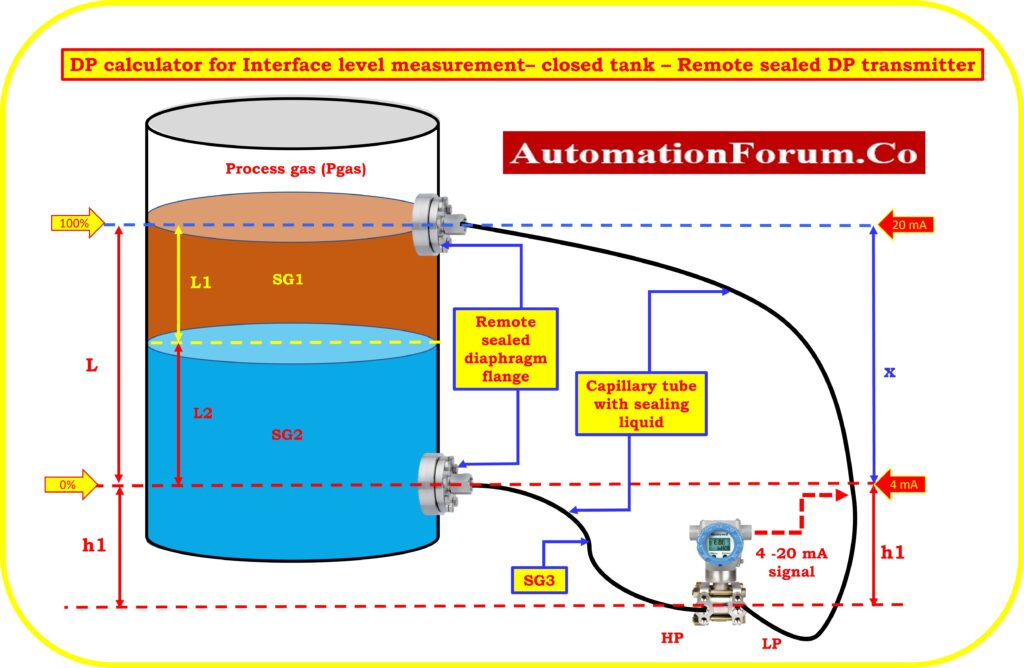# DP calculator for Interface level measurement## What is the interface liquid level measurement?

Measurement of the interface level is used to keep track of the level of two liquids with different specific gravities. When two liquids are in the same tank but can’t mix, they are separated by an emulsion layer. When two materials that don’t mix well together are put together, the liquid with the heavier molecules will sink to the bottom and the liquid with the lighter molecules will stay on top. Oil and water is a perfect example of this.

If two liquids in the same vessel or tank have different densities, or specific gravities, the denser liquid will sink to the bottom and the less dense liquid will float on top. The Interface is the place where these two liquids meet.

## How is interface level calculated?

To figure out the transmitter’s calibrated range, you should remember the following:

• The total level should be at least as high as the upper (low pressure) tap or higher.
• Either a remote seal system or a wet leg can be used to measure this.
• The height and density (SG3) of the reference level must be constant.
• At the lower range value (LRV), 4 mA, the tank is filled with lighter fluid (SG2).
• At the upper range value (URV), 20 mA, the tank is filled with the heavier fluid (SG1).

A calibrated range of 4 to 20 mA is produced by a DP transmitter, which essentially measures the difference in pressure applied to H.P. and L.P. tap.

L = Level height of the heavier liquid need to be measured

LI = Lighter liquid level need to be measured

L2 = Heavier liquid level need to be measured

x = Height distance between the DP transmitter remote sealed diaphragm flange locations (HP & LP) in tank

SG2 = Specific gravity of the heavier process liquid

SG1 = Specific gravity of the lighter process liquid

SG3= Specific gravity of the sealing liquid inside capillary of remote sealed transmitter

h1 = height between transmitter location from the minimum level of the tank

IL = Interface level

Pressure on HP side of the DP transmitter = P.HP

Pressure on the DP transmitter’s LP side = P.LP

DP = P.HP – P.LP

## LRV Calculation

For the purpose of interface level calculation, the condition in which to calculate the LRV of the differential pressure (DP) transmitter occurs when the tank must be filled with lighter liquid (SG1)

P.HP = Pgas+L*SG1+h1*SG3

P.LP = Pgas+x*SG3+h1*SG3

DP = P.HP – P.LP

LRV(4mA) = (Pgas+L*SG1+h1*SG3) – (Pgas+x*SG3+h1*SG3)

LRV(4mA) = (L*SG1) – (x*SG3)

## URV Calculation

For the purpose of interface level calculation, the condition in which to calculate the LRV of the differential pressure (DP) transmitter occurs when the tank must be filled with heavier liquid (SG2)

P.HP = Pgas+L*SG2+h1*SG3

P.LP = Pgas+x*SG3+h1*SG3

DP = P.HP – P.LP

URV(20mA) = (Pgas+L*SG2+h1*SG3) – (Pgas+x*SG3+h1*SG3)

URV(20mA) = (L*SG2) – (x*SG3)

Range of the transmitter = (L*SG1) – (x*SG3) to (L*SG2) – (x*SG3)

## DP calculator for interface Level measurement – calculate the level of the heavier liquid in the closed tank

The below calculator used to calculate LRV and URV of the transmitter